# AP Chemistry : Unit Conversions

## Example Questions

### Example Question #1 : Unit Conversions

How many moles are there in 160g of NaOH?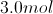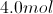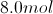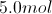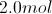Explanation:

To find the amount of moles, we need to first find the weight of each part of the compound we are looking at. Sodium (Na) weighs about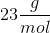.  Oxygen (O) weighs about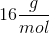.  Hydrogen weighs about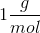.  One mole of NaOH would weigh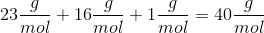.

Now we can convert grams to moles using basic stoichiometry.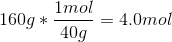### Example Question #2 : Unit Conversions

You may have heard the famous story of Archimedes and the golden crown; where the ancient Greek mathematician was challenged to determine if his King's golden crown was truly made of 100% gold. In that story Archimedes used density to determine that the crown was not 100% gold.

The density of gold is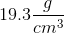In real life there are many applications for this concept that you can use in real life. For example lets say that you are in the business of selling scrap metal. You have spent a lot of time collecting a yellowish metal; but have no clue what it is, and don't wish to simply trust the judgment of the scrap metal buyer. You do know that the most likely yellow metals; given your sources for the metal are copper, brass and gold. You just don't know which it is by looking at it.

The density of copper is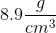The density of brass is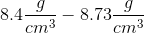the density of brass is a range because it is an alloy of copper and zinc, and can be made with different proportions of each element, whereas the density of copper is exact because it is a pure element, whose composition doesn't change (assuming none of the copper is oxidized, in which case it turns green like the statue of liberty and is no longer a yellow metal).

You have a scale and know the weight of your collected metal to be 20lbs. You take a container with water filling it to an initial volume of 40gal

Then you place your metal in the container, and the volume rises to 61.3gal. What is the identity of your metal?

1gal = 3.785L

1lb = 453.592g

Brass

Gold

Steel

Copper

None of these

Copper

Explanation:

## We know the following relationships from the information in the problem and from the definitions of the units: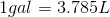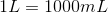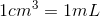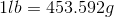## Solving the problem:

The change in volume after you submerge your metal in the container is the volume of the metal itself. Therefore: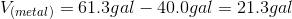Since the densities we have been given are all expressed as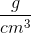we should convert our numbers into those units as shown below: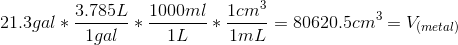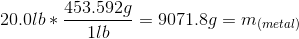Now that we have both the mass and volume of our unknown metal you can calculate the density of the metal because density is defined as: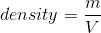So the density of our metal is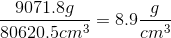Since our metal's density matches the density of copper we can now prove that the metal is pure copper.

### Example Question #3 : Unit Conversions

How many centimeters are in 14.1 inches?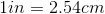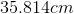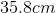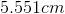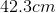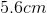Explanation: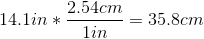Remember, there are 2.54 centimeters in every one inch. 2.54 can be multiplied by the given number of inches in order to cancel units, and convert the inches to centimeters. Answer with the same number of significant figures, as what was given in the initial number.

### Example Question #4 : Unit Conversions

How many quarts are in 13L?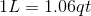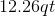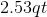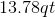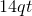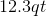Explanation: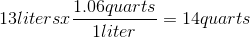Remember: there are approximately 1.06 quarts for every 1 liter; so if the given number of liters is multiplied by 1.06, it will convert the units to quarts. Make sure the answer and the given amount have the same number of significant figures.

### Example Question #5 : Unit Conversions

How many pounds is 22kg?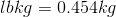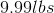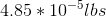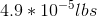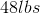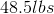Explanation: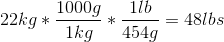Remember, the prefix "kilo" means "thousand." So there are a thousand grams, for every 1 kilogram. There are approximately 454 grams per pound, so arrange those conversion in such a way that units cancel, and pounds are the only units left. Make sure the answer and the given amount have the same number of significant figures (22 kg).

### Example Question #6 : Unit Conversions

How many meters is 8.0 yards?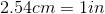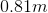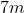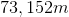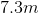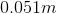Explanation: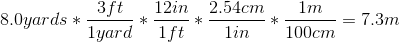This is a multi-step conversion. A U.S. to metric conversion will be needed at some point because the initial measurement is in yards (a U.S. measurement) and the answer requires meters (a metric unit). To use theconversion; change yards into inches. Once in centimeters, remember that there are 100cm in 1m; "centi" means "hundred."

### Example Question #7 : Unit Conversions

How many grams in 2 tons?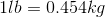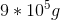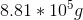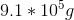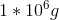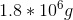Explanation: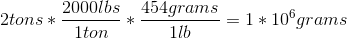The questions gives a U.S. measurement (tons) and asks for a metric unit (grams), so at some point, a U.S. measurement to metric measurement conversion will be used. There are approximately 454 grams (metric unit) per 1 pound (U.S. measurement); so tons needs to be changed into pounds in order to use that conversion. Make sure the units cancel and make sure the answer has the same number of significant figures as the given measurement.

### Example Question #8 : Unit Conversions

The density of silver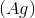is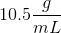. Find the mass of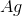that occupies 900mL of space. Express your answer in grams using the correct number of significant figures.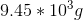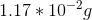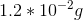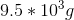Explanation:

Remember: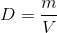In this problem we are given the density and the volume and asked to calculate the mass. Thus, by solving the above equation for mass, we get: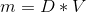Therefore, we can simply plug in our values forand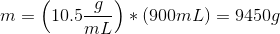However, we need to check out significant figures. 10.5 has three significant figures, and 950 has two significant figures. Therefore our answer can only have two significant figures. Therefore our answer is 9500g, written in scientific notation:### Example Question #9 : Unit Conversions

A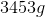sample of an unknown substance occupies a volume of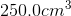. Find the density in.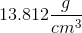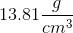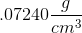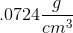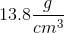Explanation:

Remember:Therefore to find density we must divide the given mass by the given volume.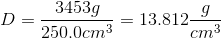However, we must remember our significant figure rules. In this case both the given values have 4 significant figures. So, our answer can only have 4 significant figures. Therefore after rounding the correct answer is.